# User-Defined Methods

## Objective Type Questions

#### Question 1

In Java, methods reside in ............... .

In Java, methods reside in classes.

#### Question 2

The number and type of arguments of a function are known as ............... .

The number and type of arguments of a function are known as function signature.

#### Question 3

The first line of function definition that tells about the type of return value along with number and type of arguments is called ............... .

The first line of function definition that tells about the type of return value along with number and type of arguments is called function prototype.

#### Question 4

Function not returning any value has return type as :

1. int
2. char
3. float
4. void

void

Reason — If no value is being returned, the return type of a function is void.

#### Question 5

A function can return ............... values.

1. 1
2. 2
3. 3
4. all the above

1

Reason — In Java, a function can return only a single value.

#### Question 6

The parameters appearing in function call statement are called ............... .

1. actual parameters
2. formal parameters
3. call parameters
4. all the above

actual parameters

Reason — The parameters that appear in method call statement are called actual parameters.

#### Question 7

The parameters appearing in function definition are called ............... .

1. actual parameters
2. formal parameters
3. call parameters
4. all the above

formal parameters

Reason — The parameters that appear in method definition are called formal parameters.

#### Question 8

The function call in which the data in actual parameters remain unchanged is known as ............... .

1. Call by Value
2. Call by Reference
3. Return by Value
4. Return by Reference

Call by Value

Reason — In Call by Value, the data of actual parameters is copied into formal parameters. Thus, actual parameters remain unchanged.

#### Question 9

The function call in which the data in actual parameters get changed is known as ............... .

1. Call by Value
2. Call by Reference
3. Return by Value
4. Return by Reference

Call by Reference

Reason — In call by reference, the called method does not create its own copy of original values, rather, it refers to the original values through the references. Thus, the called method works with the original data and any change in the values gets reflected to the data.

#### Question 10

The function that changes the state of its parameters is called ............... .

1. pure function
2. impure function
3. change function
4. none of the above

impure function

Reason — An impure function is the function that changes / modifies the state of received argument.

#### Question 11

One function, many definitions, is called ............... .

1. function enlargement
4. all the above

Reason — The process of creating overloaded functions i.e., one function with different signatures, is called function overloading.

#### Question 12

Which of the following is not an advantage of functions ?

1. it helps cope up complexity in programs
2. it makes subprograms reusable
3. it hides the implementation details
4. it offers mathematical solutions of problems

it offers mathematical solutions of problems

Reason — A function helps cope up complexity in programs, makes subprograms reusable and hides the implementation details. It does not offer mathematical solutions of problems.

#### Question 13

Name the keyword that causes the control to transfer back to the method call.

The keyword 'return' causes the control to transfer back to the method call.

#### Question 14

Name the Java keyword that indicates that a method has no return type.

The keyword 'void' indicates that a method has no return type.

## Assignment Questions

#### Question 1

What is a function ? What is its other name ? What is function prototype ?

A function is a named block of code within a class. It executes a defined set of instructions when called from another part of the program.

A function is also known as a member method.

A method/function prototype is the first line of the function definition that contains the access specifier, return type, method name and a list of parameters.

#### Question 2

What are actual and formal parameters of a function ?

The parameters that appear in the method call statement are called actual parameters.

The parameters that appear in the method definition are called formal parameters.

For example,

class ParameterDemo
{
double square(double x)    {
return Math.pow(x,2);
}

public static void main(String args[])
{
ParameterDemo obj = new ParameterDemo();
double n = 5.3;
double sq = obj.square(n);
}
}

Here, x is the formal parameter and n is the actual parameter.

#### Question 3

What is the statement specifically called that invokes a function ?

The statement that invokes a function is called method call statement.

#### Question 4

A function argument is a value returned by the function to the calling program. (T/F) ?

False.

Reason — A function argument is the value passed to the function by the method call statement at the time of method call. The value returned by the function is called the return value of the function.

#### Question 5

How many values can be returned from a function ?

A function can return only one value because as soon as return statement is executed, the execution of the method terminates and control is transferred to the method call statement.

#### Question 6

What is the condition of using a function in an expression ?

The condition of using a function in an expression is that it must return some value. The return type of the function should not be void.

#### Question 7

When a function returns a value, the entire function call can be assigned to a variable. (T/F) ?

True

Example

class Demo
{
int sum(int a, int b)   {
int sum = a + b;
return sum;
}

public static void main(String args[])  {
Demo obj = new Demo();
int ans = obj.sum(10, 20);
System.out.println(ans);
}
}

#### Question 8(a)

Identify the errors in the function skeletors given below :

float average (a, b) { }

The data type of arguments a and b is not specified.

The prototype of the function must specify the data type of the formal parameters. The correct statement is as follows:

float average (float a, float b) { }

#### Question 8(b)

Identify the errors in the function skeletors given below :

float mult (int x, y) { }

The data type of argument y is not specified.

The prototype of the function must specify the data type of the formal parameters. The correct statement is as follows:

float mult (int x, int y) { }

#### Question 8(c)

Identify the errors in the function skeletors given below :

float doer (int, float = 3.14) { }

Assignment is not allowed in function prototype. The argument list must contain the data type along with the variable name. The correct statement is as follows:

float doer (int n, float pi) { }

#### Question 9

Given the method below, write a main() method that includes everything necessary to call given method.

int thrice (int x)
{
return x * 3 ;
}

public class Methodcall
{
int thrice (int x)
{
return x * 3 ;
}

public static void main(String args[])
{
Methodcall obj = new Methodcall();
int ans;
ans = obj.thrice(5);
}
}

#### Question 10

What is the principal reason for passing arguments by value ?

The principal reason for passing arguments by value is that the actual parameters that are used to call the method cannot be modified by the called method because any changes that occurs inside the method is on the method's copy of the argument value. The original copy of the arguments remains intact.

#### Question 11

When an argument is passed by reference,

1. a variable is created in the function to hold the argument's value.
2. the function cannot access the argument's value.
3. a temporary variable is created in the calling program to hold the argument's value.
4. the function accesses the argument's original value in the calling program.

the function accesses the argument's original value in the calling program.

Reason — In call by reference, the called method does not create its own copy of original values, rather, it refers to the original values through the references. Thus, the called method works with the original data and any change in the values gets reflected to the data.

#### Question 12

What is the principal reason for passing arguments by reference ? In a function call, what all data items can be passed by reference ?

The principal reason for passing arguments by reference is that we want the modifications done by the called method to reflect back to the original variables (i.e., actual parameters). For example, a method that swaps the values of two variables. In this case, we will pass an object containing the two integers to the method so that the swapped variables are visible in the calling method.

The reference data types — objects and arrays, are passed by reference.

#### Question 13

What is the role of a return statement in a method ?

The return statement is useful in two ways:

1. As soon as a return statement is encountered, the control is returned to the calling method.
2. It is used to return a value to the method call statement.

#### Question 14

What are the three types of functions in Java?

The three types of functions in Java are-

1. Computational methods — The methods that calculate or compute some value and return the computed value. Computational methods always return a computed result. For example, Math.sqrt( ) and Math.cos( ).
2. Manipulative methods — The methods that manipulate information and return a success or failure code. Generally, if value 0 is returned, it denotes successful operation; any other number denotes failure.
3. Procedural methods — The methods that perform an action and have no explicit return value. For instance, System.out.println() method is a procedural method.

#### Question 15

Write a function that interchanges the value of two integers A and B without using any extra variable.

void swap(int a, int b)   {
a = a + b;
b = a - b;
a = a - b;
}

#### Question 16

Give the prototype of a function check which receives a character ch and an integer n and returns true or false.

boolean check(char ch, int n)

#### Question 17

Write a function that takes an int argument and doubles it. The function does not return a value.

void doubleNum(int n)   {
n = n + n;
}

#### Question 18

Differentiate between CALL by reference and CALL by value.

Call by valueCall by reference
Actual parameters are copied to formal parameters.Formal parameters refer to actual parameters.
Any changes to formal parameters are not reflected onto the actual parameters.The changes to formal parameters are reflected onto the actual parameters.
All primitive data types are passed using Call by value.All reference data types like arrays and objects of classes are passed using Call by reference.
It is used to keep original data secure and unchanged.It is used when the original data values need to be modified.

#### Question 19

Polymorphism is the property by which the same message can be sent to objects of several different classes and each object can respond in a different way depending on its class.

In function overloading, a function name has several definitions in the same scope. These function definitions are distinguished by their signatures. The same function behaves differently with different number and types of arguments.

Let us consider the given example in which the function perimeter() is overloaded. In each function, the perimeter of a different shape (square, rectangle and trapezium) is calculated.

public class Perimeter
{
public double perimeter(double s)
{
double p = 4 * s;
return p;
}

public double perimeter(double l,
double b)
{
double p = 2 * (l + b);
return p;
}

public double perimeter(double a,
double b,
double c,
double d)
{
double p = a + b + c + d;
return p;
}
}

#### Question 20

A function name having several definitions in the same scope that are differentiable by the number or types of their arguments, is said to be an overloaded function. The process of creating overloaded functions is called function overloading.

#### Question 21

1. It implements the object oriented concept of Polymorphism.
2. It reduces the number of com­parisons in a program and thereby makes the program run faster.

#### Question 22

What is the role of a function's signature in disambiguation process?

The key to function overloading is a function's signature. The signatures can differ in the number of arguments or in the type of arguments, or both. To overload a function name, we need to declare and define all the functions with the same name but different signatures, separately.

At the time of function call, the compiler matches the signature of overloaded function with the signature of the method call statement and executes the function with the matching signature.

#### Question 23

What factors make two definitions with the same function name significantly different ?

The following factors make two definitions with the same function name significantly different:

1. The number of arguments
2. The type of arguments

#### Question 24

How does the use of constant suffixes help avoid ambiguity when an overloaded function is called ?

Sometimes, there might be ambiguity between float and double values or int or long values.

For instance, if you want to invoke the function with following declaration: void prnsqr(double d) with the value 1.24. This value may also be assumed to be float as well as double.

To avoid such ambiguity, we can use constant suffixes (F, L, D, etc.) to distinguish between such values as these greatly help in indicating which overloaded function should be called.

An ordinary floating constant (312.32) has the double type, while adding the F suffix (312.32 F) makes it a float. The suffix L (312.32L) makes it a long double. Similarly, suffix D or d makes it a double.

#### Question 25

Two methods cannot have the same name in Java. (True/False)

False

Reason — Two or more methods can have the same name in Java if the functions are overloaded. For function overloading, many function definitions can have the same name but they must have different signatures.

#### Question 26

We can overload methods with differences only in their return type. (True/False)

False

Reason — We can't overload methods with differences only in their return type. The methods must have distinct signatures as well.

#### Question 27

Members of a class specified as private are accessible only to the methods of the class. (True/False)

True

Reason — The data members declared as 'private' can only be accessed by the member methods of the same class.

#### Question 28

A method declared as static cannot access non-static class members. (True/False)

True

Reason — A method declared as static can only access static class members.

#### Question 29

A static class method can be invoked by simply using the name of the method alone. (True/False)

False

Reason — A method declared as static can be invoked by using the syntax <class name>.<method name>. For example, in Math.pow() function, Math is the name of the class and pow() is its static function.

#### Question 30

Which of the following function-definitions are overloading the method given below :

int sum(int x, int y) {}
1. int sum(int x, int y, int z) { }
2. float sum(int x, int y) { }
3. int sum (float x, float y) { }
4. int sum (int a, int b) { }
5. float sum(int x, int y, float z) { }

1. int sum(int x, int y, int z) { }
2. int sum (float x, float y) { }
3. float sum(int x, int y, float z) { }

Reason — Function prototypes 1,3 and 5 have different signatures. Thus, they are overloading the function sum(). Prototypes 2 and 4 have same signatures as both are taking two int arguments, which will generate compile time error.

#### Question 31

What is the role of void keyword in declaring functions ?

The keyword 'void' signifies that the method doesn't return a value to the calling method.

#### Question 32

How is call-by-value way of function invoking different from call-by-reference way ? Give appropriate examples supporting your answer.

In call by value, the called function creates its own work copy for the passed parameters and copies the passed values in it. Any changes that take place remain in the work copy and the original data remains intact.

In call by reference, the called function receives the reference to the passed parameters and through these references, it accesses the original data. Any changes that take place are reflected in the original data.

This can be clearly understood by the example given below:

public class DemoFnCalls
{
public static int x = 10 ;
public static int y = 20 ;

public void DemoTest()  {
System.out.println("Values initially. x =" + x + ",  y =" + y);
swapCallByValue(x, y);
System.out.println("Values after swapCallByValue method. x ="
+ x + ", y =" + y) ;
DemoFnCalls object1 = new DemoFnCalls( ) ;
swapCallByRef(object1);
System.out.println ("Values after swapCallByRef method. x ="
+ x + ", y =" + y) ;
}

public static void swapCallByValue(int a, int b)  {
int tmp;
tmp = a;
a = b;
b = tmp;
System.out.println("Values inside swapCallByValue method. x ="
+ a + ", y = " + b);
}

public static void swapCallByRef(DemoFnCalls obj)   {
int tmp;
tmp = obj.x;
obj.x = obj.y;
obj.y = tmp;
System.out.println("Values inside swapCallByRef method. x ="
+ obj.x + ", y =" + obj.y);
}
}

#### Question 33

What is the output of the following program ? Justify your answer.

class Check	{
public static void chg (String (nm) )   {
nm = "Aamna" ;	//  copy "Aamna" to nm
}
public void test( ) {
String name= "Julius";
System.out.println (name);
chg(name);
System.out.println(name);
}
}

The program has a syntax error in the chg method parameter definition. The correct syntax would be public static void chg(String nm), without the parentheses around nm.

Assuming this error is fixed, then the output of the program will be as follows:

Julius
Julius

##### Explanation

In Java, String objects are treated differently because String objects are immutable i.e., once instantiated, they cannot change. When chg(name) is called from test() method, name is passed by reference to nm i.e., both name and nm variable point to the same memory location containing the value "Julius" as shown in the figure below: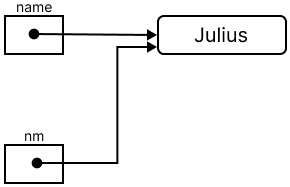Inside chg() method, when the statement nm = "Aamna" ; is executed, the immutable nature of Strings comes into play. A new String object containing the value "Aamna" is created and a reference to this new object is assigned to nm as shown in the figure below: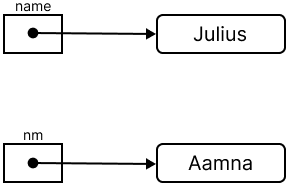The variable name still holds the reference to the memory location containing the value "Julius". After chg() finishes execution and control comes back to test() method, the value of name is printed as "Julius" on the console.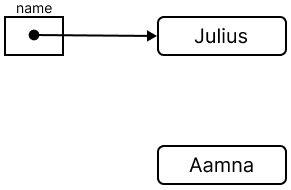#### Question 34

Write a function that takes two char arguments and returns 0 if both the arguments are equal. The function returns -1 if the first argument is smaller than the second and 1 if the second argument is smaller than the first.

class KboatCompareChar
{
int compareChar(char c1, char c2)   {

int ret;

if(c1 == c2)
ret = 0;
else if(c1 < c2)
ret = -1;
else
ret = 1;

return ret;
}

}
##### Output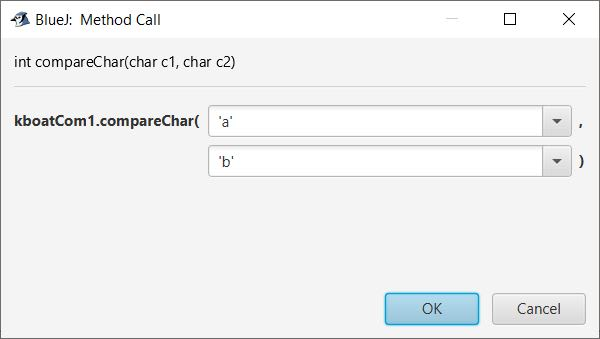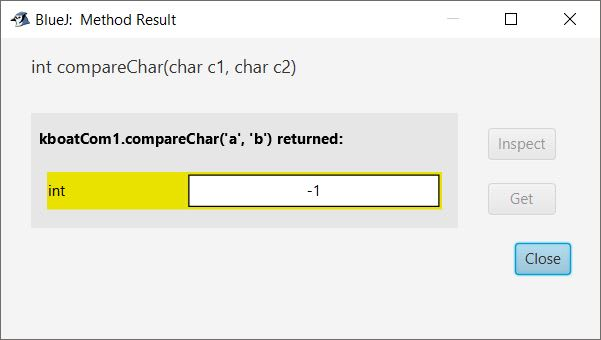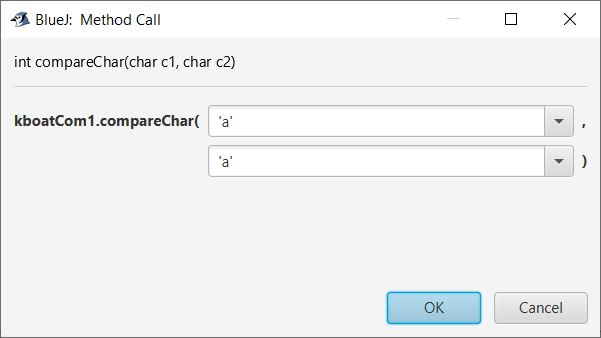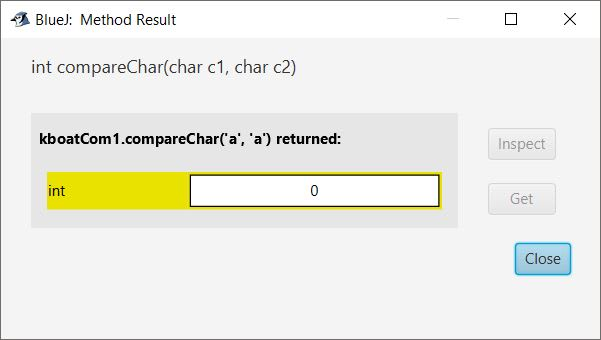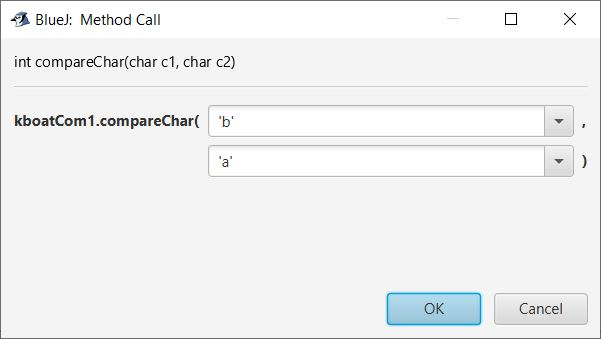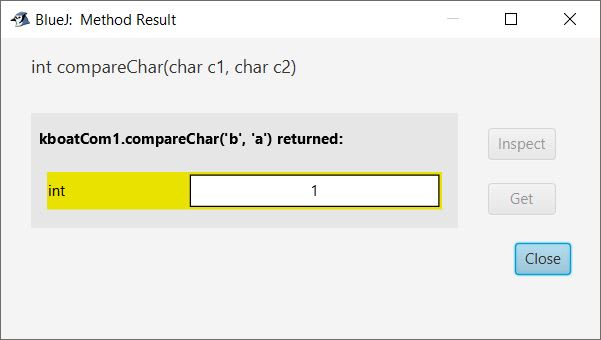#### Question 35

Write a complete Java program that invokes a function satis() to find whether four integers a, b, c, d sent to satis( ) satisfy the equation a3 + b3 + c3 = d3 or not. The function satis( ) returns 0 if the above equation is satisfied with the given four numbers otherwise it returns -1.

import java.util.Scanner;

class KboatCheckEquation
{
int satis(int p,
int q,
int r,
int s) {
int res;

double lhs = Math.pow(p,3)
+ Math.pow(q,3)
+ Math.pow(r,3);

double rhs = Math.pow(s,3);

if(lhs == rhs)
res = 0;
else
res = -1;

return res;
}

public static void main(String args[])
{
KboatCheckEquation obj = new KboatCheckEquation();

Scanner in = new Scanner(System.in);
System.out.print("Enter a: " );
int a = in.nextInt();
System.out.print("Enter b: " );
int b = in.nextInt();
System.out.print("Enter c: " );
int c = in.nextInt();
System.out.print("Enter d: " );
int d = in.nextInt();

int res = obj.satis(a, b, c, d);

if(res == 0)
System.out.println("Equation satisfied");
else
System.out.println("Equation not satisfied");
}
}
##### Output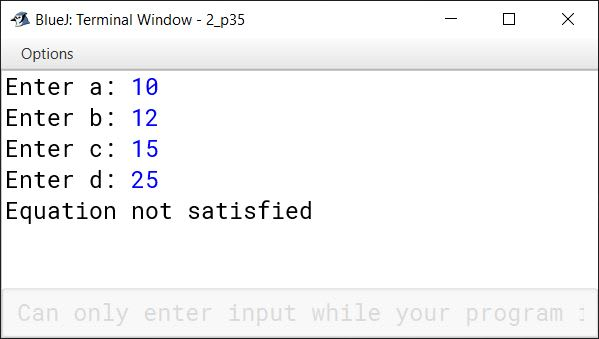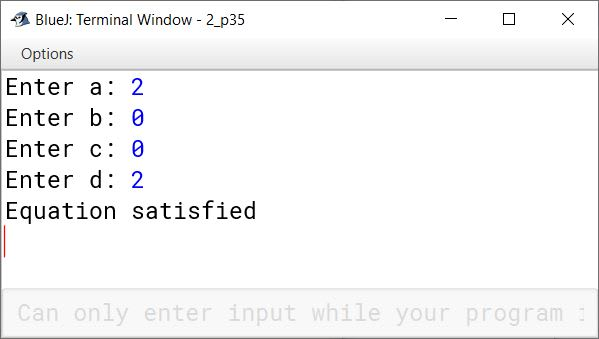#### Question 36

Write a program that uses a method power( ) to raise a number m to power n. The method takes int values for m and n and returns the result correctly. Use a default value of 2 for n to make the function calculate squares when this argument is omitted. Write a main( ) method to get the value of m and n to display the calculated result.

import java.util.Scanner;

public class KboatCalcPower
{
public double power(int m)   {
double pow = Math.pow(m,2);
return pow;
}

public double power(int m, int n)   {
double pow = Math.pow(m,n);
return pow;
}

public static void main(String args[])  {

KboatCalcPower obj = new KboatCalcPower();

Scanner in = new Scanner(System.in);
System.out.print("Enter m: ");
int m = in.nextInt();
System.out.print("Enter n: ");
int n = in.nextInt();

double res = obj.power(m,n);
System.out.println("m^n = " + res);

res = obj.power(m);
System.out.println("Omitting n");
System.out.println("m^2 = " + res);
}
}
##### Output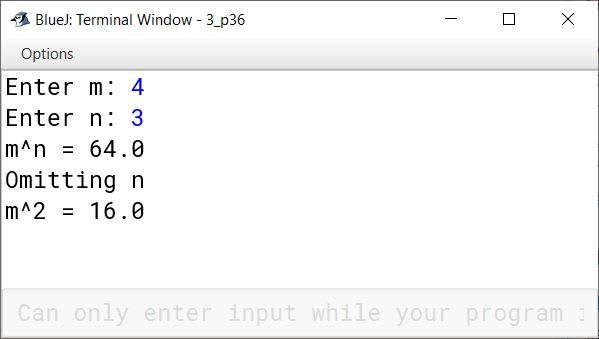#### Question 37

How does the compiler interpret more than one definitions having same name ? What steps does it follow to distinguish these ?

The compiler interprets more than one definitions having same name by matching the signature in the function definitions with the arguments given in the method call statement.

When a function name is declared more than once in a program, the compiler will interpret the second and subsequent declarations as follows:

1. If the signatures of subsequent functions match the previous function's, then the second is treated as a re-declaration of the first and is flagged at compile time as an error.
2. If the signatures of the two functions match exactly but the return types differ, the second declaration is treated as an erroneous re-declaration of the first and is flagged at compile time as an error. For example:
float square(float f) {...} and double square(float x) will be treated as an error.
Functions with the same signature and same name but different return types are not allowed in Java. We can have different return types, but only if the signatures are also different :
float square (float f) and double square (double d)
3. If the signatures of the two functions differ in either the number or type of their arguments, the two functions are considered to be overloaded.

#### Question 38

Discuss how the best match is found when a call to an overloaded method is encountered. Give example(s) to support your answer.

When an overloaded function is called, the compiler matches the signature in the function definitions with the arguments given in the method call statement and executes the function once the match is found.

To avoid ambiguity, we can use constant suffixes (F, L, D, etc.) to distinguish between values passed as arguments. These greatly help in indicating which overloaded function should be called. For example, an ordinary floating constant (e.g., 312.32) has the double type, while adding the F suffix (e.g., 312.32 F) makes it a float.

For example, the following code overloads a function perimeter() which calculates the perimeter of square, rectangle and trapezium.

public class Perimeter
{
public double perimeter(double s)
{
double p = 4 * s;
return p;
}

public double perimeter(double l,
double b)
{
double p = 2 * (l + b);
return p;
}

public double perimeter(double a,
double b,
double c,
double d)
{
double p = a + b + c + d;
return p;
}

public static void main(String args[])  {
Perimeter obj = new Perimeter();
double areaSquare = obj.perimeter(5.8);
double areaRectangle = obj.perimeter(10.5, 4.5);
double areaTrapezium = obj.perimeter(4, 10, 7, 15);
System.out.println(areaSquare + " "
+ areaRectangle + " "
+ areaTrapezium);
}
}
##### Output
23.2 30.0 36.0


#### Question 39

Design a class to overload a function area( ) as follows :

(i) double area(double a, double b, double c) with three double arguments, returns the area of a scalene triangle using the formula :

$\text{area} = \sqrt{s(s-a)(s-b)(s-c)}$

where $s = \dfrac{a +b + c}{2}$

(ii) double area(int a, int b, int height) with three integer arguments, returns the area of a trapezium using the formula :

area = $\dfrac{1}{2}$ height(a + b)

(iii) double area(double diagonal1, double diagonal2) with two double arguments, returns the area of a rhombus using the formula :

area = $\dfrac{1}{2}$ (diagonal1 x diagonal2)

import java.util.Scanner;

{
double area(double a, double b, double c) {
double s = (a + b + c) / 2;
double x = s * (s-a) * (s-b) * (s-c);
double result = Math.sqrt(x);
return result;
}

double area (int a, int b, int height) {
double result = (1.0 / 2.0) * height * (a + b);
return result;
}

double area (double diagonal1, double diagonal2) {
double result = 1.0 / 2.0 * diagonal1 * diagonal2;
return result;
}
}
##### Output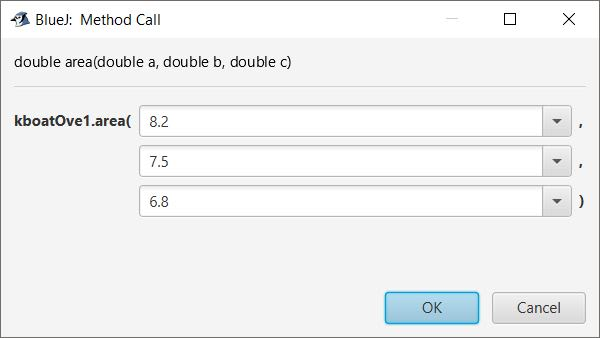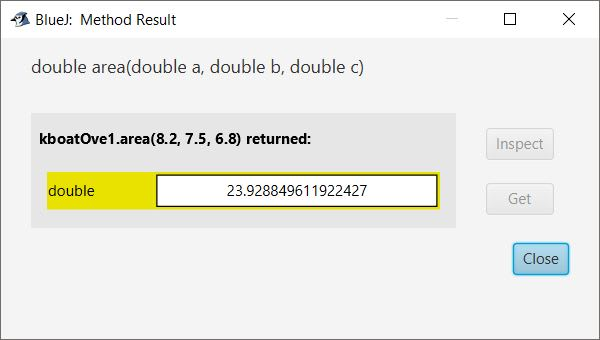#### Question 40

What is the output of the following program?

class AllStatic
{
static int m = 0 ;
static int n = 0 ;
public static void main(String[ ] args)
{
int m = 10;
int x = 20;
{
int n = 30 ;
System.out.println("m + n =" + m + n) ;
check(5) ;
}
x = m + n;
System.out.println("x =" + x) ;
}
public static void check int  k ;
{
int m = 5 ;
n = k;
System.out.println("m is " + m) ;
System.out.println("n is " + n) ;
}
}

The given code generates an error due to wrong method prototype — public static void check int k ;

The correct syntax of the method will be —

public static void check (int k)

Assuming the method prototype was correct, the following output will be generated:

m + n = 1030
m is 5
n is 5
x = 15

##### Explanation

Initially, m = 10 and n = 30.

The value 5 is passed by value to the function check(). The function check() declares a local variable m whose value is 5. The value of n is modified to 5. Both the values (m and n) are printed and then the control goes back to the statement following the method call.

The value of x becomes 15 (since m = 10 and n = 5). Inside the main(), the value of local variable m is 10 and n is 5.

#### Question 41

What is the output of the following code ?

void func(String s)	{
String s = s1 + "xyz" ;
System.out.println("s1 =" + s1) ;
System.out.println("s =" + s) ;
}